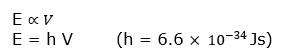# Planck Quantum Theory And Photoelectric effect

## PLANCK’S QUANTUM THEORY: -

This theory was given by plank to explain the phenomenon of black body radiation and photoelectric effect
This theory has following traits: -
a) The radiation energy emitted or observed in the form of small packets of energy. Such packets are known as quantum or photon.
b) Energy of each photon is directly proportional to frequency of radiation.Where ‘h’ is planck’s constant.

## PHOTOELECTRIC EFFECT:-

It was discovered by Hertz.
When a beam of light of certain frequency (threshold frequency) strikes the metal surface, electrons are emitted or ejected from the metal surface. this phenomenon is known as photoelectric effect.

### Observations in Photoelectric Effect:-

1)For each metal there is a characteristic minimum frequency below which photoelectric effect is not observed. This is called threshold frequency.
If frequency of light is less than the threshold frequency there is no ejection of electrons no matter how long it falls on surface or how high is its intensity
2) The kinetic Energy of electrons emitted is directly proportional to frequency of striking photons & independent of their intensity
3) The no. of electrons that are ejected per second from metal surface depends upon intensity of striking radiations and doesn’t depend upon their frequency.

### Explanation of Photoelectric Effect:-

Einstein could explain photoelectric effect using Plank’s Quantum theory as follows: -
a) Photoelectrons are ejected only when incident light has threshold frequency. (hν – hν0)
b) If frequency of incident light is more than threshold frequency then the excess energy is imparted to electrons in the form of kinetic energy. (E – W i. e. ½ mv2)
E = W + ½ m v2
hv = hvo + ½ mv2
c) Greater he frequency of incident light, greater the kinetic energy of e-.
d) Greater the intensity of light more the no. of electrons ejected.

## BLACK BODY RADIATIONS:-

The ideal body which emit and absorbs all the frequencies is called Black body and the radiation emitted by such body is called Black body radiation.
The exact frequency distribution of emitted radiations from black body depends only on it’s temperature.

Question 1
Electrons are emitted with 0 velocities from metal surface when exposed to radiation of wavelength 6800 Ao. Calculate Vo & W.
Solution
Given ? = 6800 Ao. or 6800x 10-10 m = 6.8 x 10-7m
C = 3 x 108 m/s

Also ? = c/v or v = c/ ? Therefore putting the values in the equation, we get

=3 x 108 / 6.8 x 10-7m = 4.41 x 1014 s-1
Hence, work function (W0) of the metal = h?0
= (6.626 × 10-34 Js) (4.41 × 1014 s-1)
= 2.922 × 10-19 J
Question 2
A proton of wavelength 400 nm strikes metal surface. The electrons are ejected with velocity 5.85 × 105 m/s. Calculate min. energy required to remove electron from metal surface. (Mass of electron = 9.1 × 10-31 kg)
Solution
Given:-
λ = 400 nm
Velocity = 5.85 × 105 m/s
C = νλ

3 × 108 m/s = ν. 400 × 10(-9) m
or
ν= 7.5 × 10(14) m
Now E = hν
= 6 × 10-34 × 7.5 × 1014
= 45 ×10-20 J
Kinetic Energy of Electron =1/2 mv2
=.5 × 9.1 × 10-31 × 5.85 × 105 × 5.85 × 105
=15.57 ×10-20 J
Min Energy = 45 ×10-20 J - 15.57 ×10-20 J = 29.43 ×10-20 J